# Absolute Convergence

Previous: Proof of the Ratio Test

Next: Absolute Convergence Implies Convergence

The ratio test requires the idea of absolute convergence. Given any infinite series Σak, we can introduce the corresponding serieswhose terms are the absolute values of the original series. We can explore whether this corresponding series converges, leading us to the following definition.

Definition: Absolute Convergence
The infinite series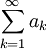is absolutely convergent if the series of absolute values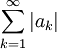is convergent.

A few simple examples demonstrate the concept of absolute convergence.

### Example: Convergent p-Series

The infinite series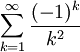is absolutely convergent because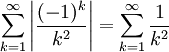is a convergent p-series (p =2).

### Example: Harmonic Series

The infinite seriesis convergent (by the alternating series test), but is not absolutely convergent because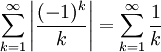is the infamous harmonic series, which is not a convergent series.

It is possible for a series to be convergent, but not absolutely convergent (such series are termed conditionally convergent, but we do not need this definition for our purposes).

Previous: Proof of the Ratio Test

Next: Absolute Convergence Implies Convergence